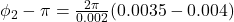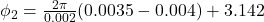## A spherical wave with a wavelength of 2.0 mm is emitted from the origin. At one instant of time, the phase at rrr = 4.0 mm is πradπrad. At t

Question

A spherical wave with a wavelength of 2.0 mm is emitted from the origin. At one instant of time, the phase at rrr = 4.0 mm is πradπrad. At that instant, what is the phase at rrr_1 = 3.5 mm ? Express your answer to two significant figures and include the appropriate units.

in progress 0
1 week 2021-09-05T07:07:30+00:00 1 Answers 0 views 0

1. Complete Question

A spherical wave with a wavelength of 2.0 mm is emitted from the origin. At one instant of time, the phase at r_1 = 4.0 mm is π rad. At that instant, what is the phase at r_2 = 3.5 mm ? Express your answer to two significant figures and include the appropriate units.

The phase at the second point is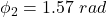Explanation:

From the question we are told that

The wavelength of the spherical wave is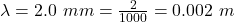The first radius  is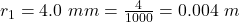The phase at that instant is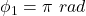The second radius is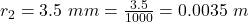Generally the phase difference is mathematically represented as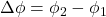this can also be expressed as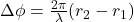So we have that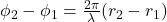substituting values